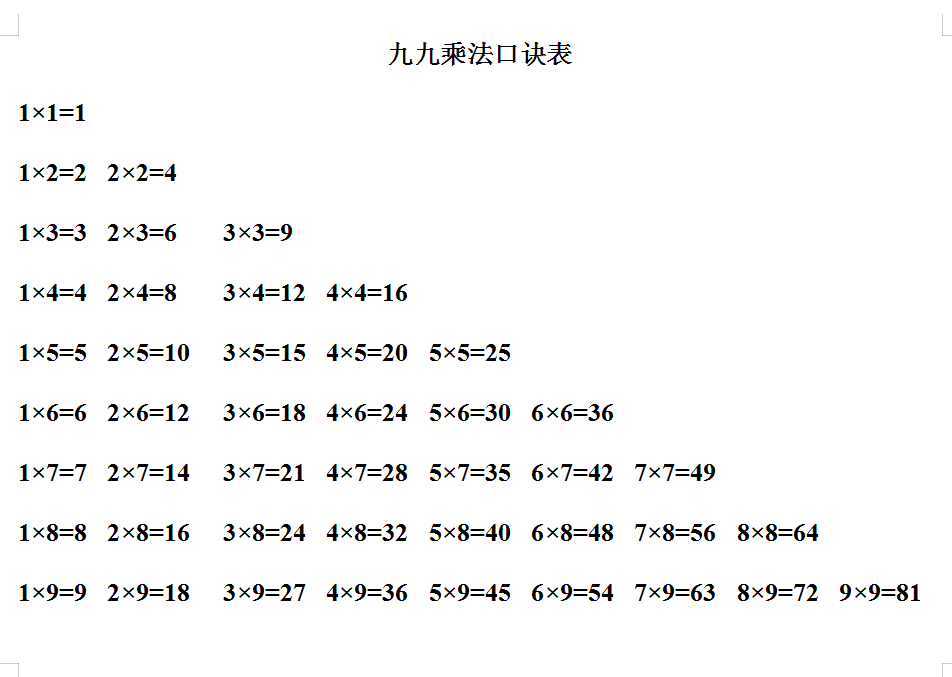1×1=1

1×2=2  2×2=4
1×3=3  2×3=6    3×3=9
1×4=4  2×4=8    3×4=12  4×4=16